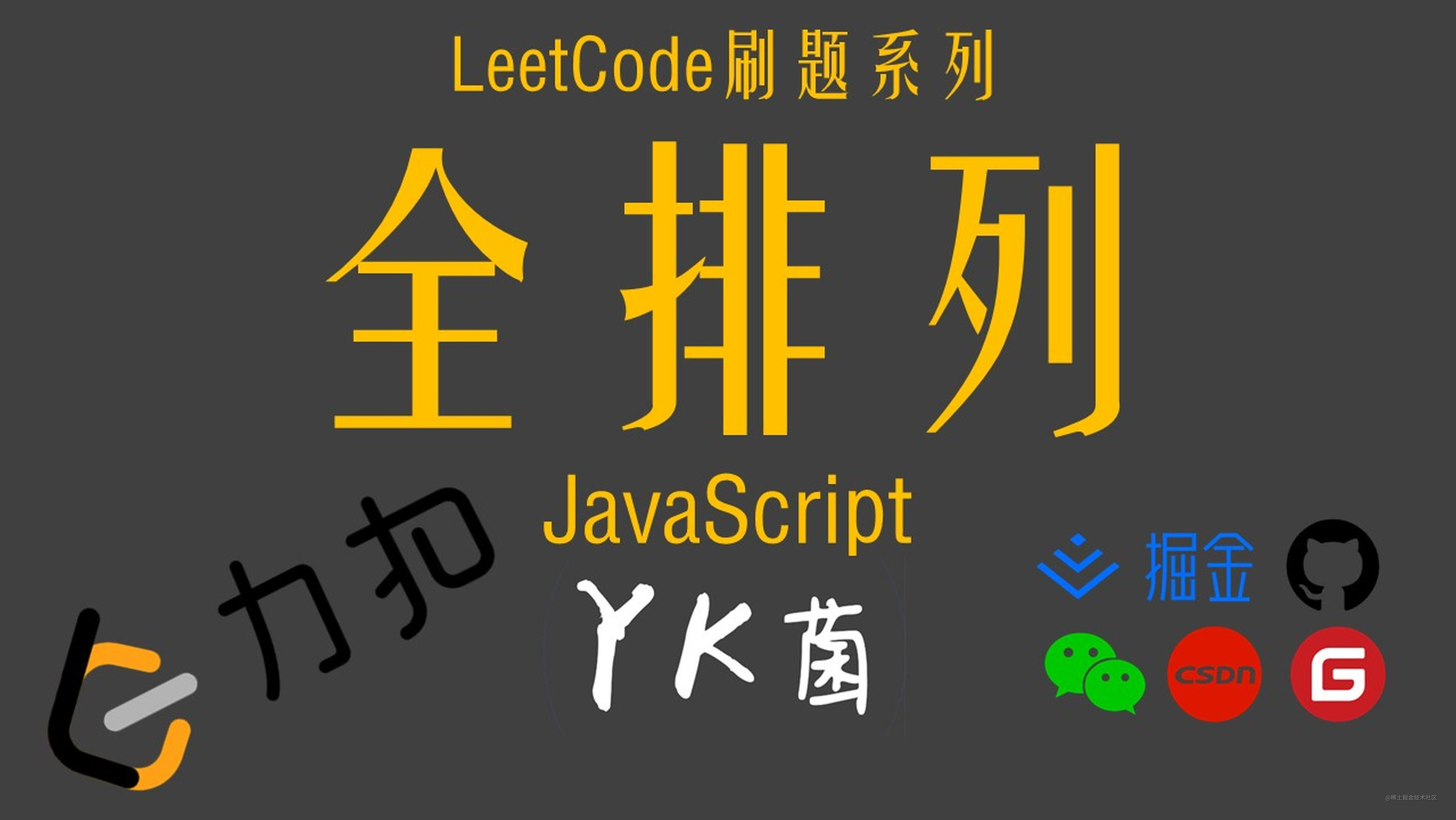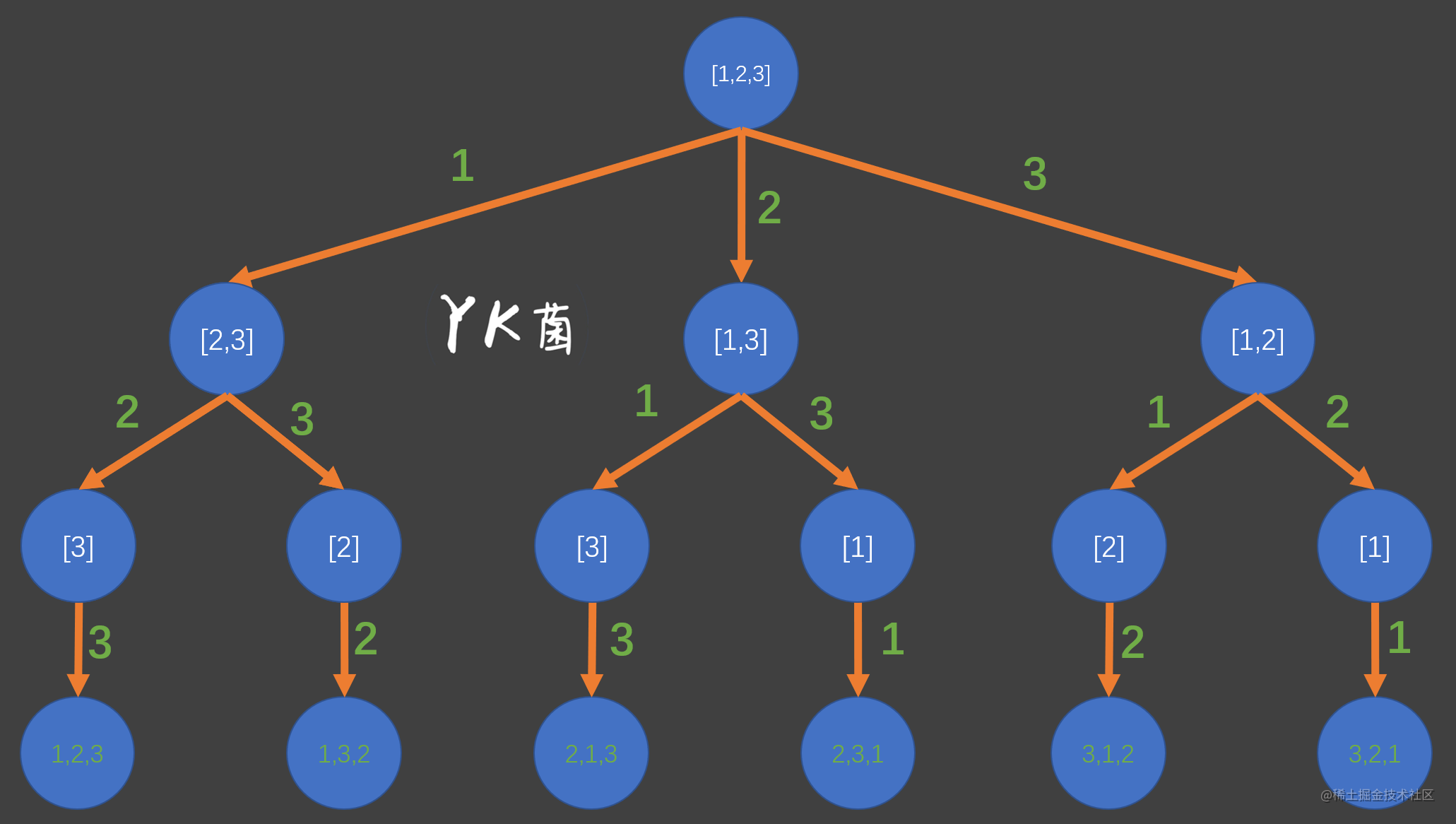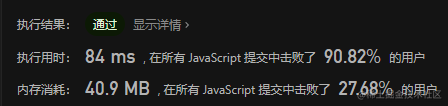# 【LeetCode】全排列 - 回溯算法 - JavaScript描述## 46. 全排列### 【解法】回溯

``````/**
* @param {number[]} nums
* @return {number[][]}
*/
var permute = function (nums) {
// 保存结果数组，保存每个路径（排列）
const result = [];
// 调用回溯函数，传入参数
backtracking(nums, nums.length, [], []);
// 返回结果数组
return result;

// 定义回溯递归函数，传入数组，长度，节点是否被使用过的数组
// used 用来标记节点是否被用过 path 用来存储路径，定义为一个栈
function backtracking(nums, len, used, path) {
// 递归出口
// 如果到达叶子节点，将路径推入结果数组，并返回
if (path.length === len) {
result.push([...path]);
return;
}
// 遍历候选字符
for (let i = 0; i < len; i++) {
// 使用过就下一轮循环
if (!used[i]) {
// undefind和fasle都会进来
// 这里说明这个数还没有被使用，入栈path
path.push(nums[i]);
// 标记这个数被使用过了
used[i] = true;
// 开始进行递归
backtracking(nums, len, used, path);
// 回溯【状态重置】撤销之前的操作
path.pop();
used[i] = false;
}
}
}
};
``````